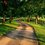# [Polyhedral Combinatorics] Euler's Formula for Convex Polyhedra using Inductive Proof

If we imagine a light source placed near one of the faces of the polyhedron above a plane, the shadows of the polyhedron edges form a planar graph embedded in the plane. This resulting figure in the plane has $V$ vertices, $E$ edges, but $F-1$ faces, because the face that was closest to the light source becomes the outside face of this figure. In this way, every convex polyhedron can be projected onto the plane without the edges crossing, hence can be represented as a planar graph.

Therefore, by showing that a connected planar graph with V vertices, E edges, and F faces satisfy the equation $V-E+F =1$, we will have shown that Euler's formula $V-E+f=2$ holds for a convex polyhedron.

Let statement $P(n)$: All connected planar graphs with E edges satisfies $V-E+F =1$.

$(1)$ Base case: $n=1=E$

There exist only one planar graph that has one edge. This graph has two vertices, one edge, and no face. Since $2-1+0 = 1$, it satisfies the formula. So $P(1)$ is true.

$(2)$ Assume $P(k)$ is true. This means that every connected planar graph with $k$ edges satisfies the formula. Now we want to show that $P(k+1)$ is also true. Consider a connected planar graph $\Gamma$ with $k+1$ edges, and say $\Gamma$ has $v$ vertices and $f$ faces. We want to show that $\Gamma$ satisfies the formula $v-(k+1)+f =1$. We can do this by removing a carefully chosen edge from $\Gamma$ to leave a connected planar graph with $k$ edges, then use $P(k)$ to show $P(k+1)$. In the case that $\Gamma$ has at least one face, that is, $f\geq 1$, we can remove one edge of this face, and the remaining graph will still be connected with $k$ edges, $v$ vertices, and $f-1$ faces. Since we assumed $P(k)$ to be true, this remaining graph will satisfy $v-k+(f-1) = 1$, and this gives $v-(k+1)+f=1$ as we required. The other case is when $\Gamma$ has no faces at all, so $f=0$. This means that there exists at least one vertex that is joined by an edge that connects to only one other vertex. If we remove this edge, our vertex will also be removed. So the resulting graph will have $v-1$ vertices, $k$ edges, and no faces. This graph also satisfies the formula by $P(n)$, which mans that $(v-1) -k+0 =1$, hence $v-(k+1)+0 =1$. However, in this case the resulting graph is not a connected planar graph anymore, but rather, a disjoint union of two graphs. Therefore, we do not need to include this case.

Therefore, we have shown that $P(n+1)$ is true whenever $P(n)$ is true.

Since $P(n)$ is true for $n=1$, and $P(n) \Rightarrow P(n+1)$, by Principle of Mathematical Induction we conclude that every connected planar graph with V vertices, E edges and F faces satisfies $V-E+F=1$. Since every convex polyhedron can be represented as a planar graph with one less face, we conclude that Euler's formula $V-E+f=2$ holds for any convex polyhedron.Note by Bright Glow
3 years, 2 months ago

This discussion board is a place to discuss our Daily Challenges and the math and science related to those challenges. Explanations are more than just a solution — they should explain the steps and thinking strategies that you used to obtain the solution. Comments should further the discussion of math and science.

When posting on Brilliant:

• Use the emojis to react to an explanation, whether you're congratulating a job well done , or just really confused .
• Ask specific questions about the challenge or the steps in somebody's explanation. Well-posed questions can add a lot to the discussion, but posting "I don't understand!" doesn't help anyone.
• Try to contribute something new to the discussion, whether it is an extension, generalization or other idea related to the challenge.

MarkdownAppears as
*italics* or _italics_ italics
**bold** or __bold__ bold
- bulleted- list
• bulleted
• list
1. numbered2. list
1. numbered
2. list
Note: you must add a full line of space before and after lists for them to show up correctly
paragraph 1paragraph 2

paragraph 1

paragraph 2

[example link](https://brilliant.org)example link
> This is a quote
This is a quote
    # I indented these lines
# 4 spaces, and now they show
# up as a code block.

print "hello world"
# I indented these lines
# 4 spaces, and now they show
# up as a code block.

print "hello world"
MathAppears as
Remember to wrap math in $$ ... $$ or $ ... $ to ensure proper formatting.
2 \times 3 $2 \times 3$
2^{34} $2^{34}$
a_{i-1} $a_{i-1}$
\frac{2}{3} $\frac{2}{3}$
\sqrt{2} $\sqrt{2}$
\sum_{i=1}^3 $\sum_{i=1}^3$
\sin \theta $\sin \theta$
\boxed{123} $\boxed{123}$